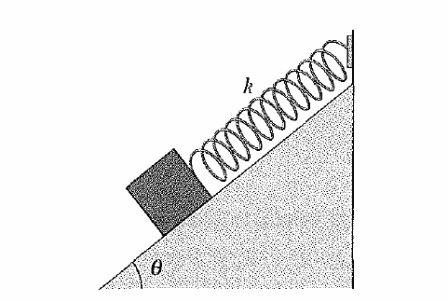# Block,spring and an inclined plane.In figure, a block weighing $14.0 N$, which can slide without friction on an incline at angle $\theta = 40.0°$, is connected to the top of the incline by a massless spring of un stretched length $0.450 m$ and spring constant $120 N/m$. How far from the top of the incline is the block's equilibrium point?

Liked it? Try more here

×